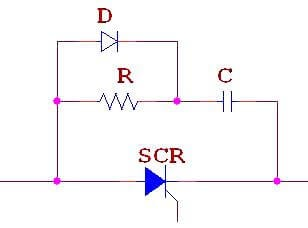# How Snubber Circuit works ?

The Snubber circuit is one type of dv/dt protection circuit of the thyristor.

With the help of snubber circuit, the false turn-on of a thyristor due to large dv/dt can be prevented.RC Snubber Circuit for SCR dv/dt Protection:

This type of snubber circuit consists of a series combination of resistance R and Capacitance C in parallel with a SCR.

• When a reverse voltage is applied, commutation process is initiated and the forward current flow through SCR approaches zero.
• Due to the inductance, current continuous to flow due to sweeping of charge carries at the external junctions.
• When it reaches a peak value it cannot be further supported by the charge carriers and falls very quickly to zero. This causes a voltage spike with the value of L(di/dt).
• Also when the supply is closed to the circuit( in the above figure say the switch S is closed), sudden voltage appears across SCR.
• Now, as the thyristor current is zero it can be considered as an open switch.
• At this moment, the capacitor C behaves  like a short-circuit and therefore voltage across the SCR is zero.
• With the passage of time capacitor C gets charged at a slow rate such that dv/dt across the capacitor and therefore across SCR is less than the specified maximum dv/dt rating of the device.
• Thus the capacitor protects the SCR against high voltages and high dv/dt.

Based on the above discussion we can say that simply a Capacitor C is sufficient to protect the SCR against dv/dt false triggering.

Then what is the purpose of resistance R?

• In the RC snubber circuit, the resistance R limits the discharge current of capacitor at the instant of firing of SCR.
• Before SCR is fired, capacitor C charges to full voltage V.
• If SCR is fired, when the capacitor voltage is maximum, it discharges through the local path formed by capacitor C, Resistance R and SCR.
• During this time, if the resistance R is not included in the circuit, the discharge current will be high and consequently may damage the SCR due to large di/dt.
• Thus the Resistance R in the snubber circuit reduces the discharge current of the capacitor C and thus protect the SCR against large di/dt.

In actual practice, R, C and the load current parameters should be such that

1. dv/dt across C during its charging is less than the specified dv/dt rating of the SCR
2. Discharge current at the turn ON of the SCR is within reasonable limits.

Normally R,S and load circuit parameters form an undamped circuit so that dv/dt is limited to acceptable values.In some RC snubber circuits, a diode D used to connect in parallel with the resistor R. It is used for the purpose of bypass and thus giving improved dv/vt protection.

Don't Miss Our Updates
Be the first to get exclusive content straight to your email.
We promise not to spam you. You can unsubscribe at any time.